Geometric distribution

(diff) ← Older revision | Latest revision (diff) | Newer revision → (diff)

The distribution of a discrete random variable assuming non-negative integral valueswith probabilities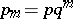, where the distribution parameter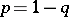is a number in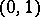. The characteristic function is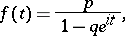the mathematical expectation is; the variance is; the generating function is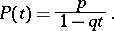Figure: g044230a

A geometric distribution of probability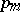.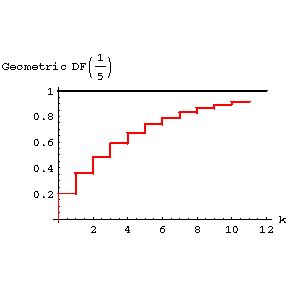Figure: g044230b

The distribution function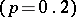.

The random variable equal to the number of independent trials prior to the first successful outcome with a probability of successand a probability of failurehas a geometric distribution. The name originates from the geometric progression which generates such a distribution.

How to Cite This Entry:
Geometric distribution. Encyclopedia of Mathematics. URL: http://www.encyclopediaofmath.org/index.php?title=Geometric_distribution&oldid=12864
This article was adapted from an original article by V.M. Kalinin (originator), which appeared in Encyclopedia of Mathematics - ISBN 1402006098. See original article